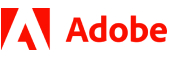New update is available. Click here to update.
Topics

# Valid Pairs

Easy0/40
Average time to solve is 22m+2 more companies

## Problem statement

You are given an array 'ARR' of 'N' integers and two integers 'K' and 'M'.

You need to return true if the given array can be divided into pairs such that the sum of every pair gives remainder 'M' when divided by 'K'. Otherwise, you need to return false.

For example:

``````If the given array is [2, 1, 5, 7] and K = 9 and M = 3. Then you need to return true because we can divide the array into two pairs, i.e (2, 1) and (5, 7) whose sums are 3 and 12, which when divided by 9 gives remainder 3, thus it is possible to divide the given array into pairs.
``````

Note:

``````Every element of the array should contribute to only one pair, i.e if the array is [3, 0, 0] and K = 2 and M = 1, then you need to return false, as element 3 will make a pair with any one of the 0.
``````
Detailed explanation ( Input/output format, Notes, Images )
Sample Input 1:
``````1
4
2 1 5 7
9 3
``````
Sample Output 1:
``````True
``````
Explanation for input 1:
``````Pairs will be {2,1} and {5,7} whose sums are 3 and 12 which will give remainder 3 when divided by 9.
``````
Sample Input 2:
``````1
5
6 6 3 0 0
9 3
``````
Sample Output 2:
``````False
``````
Explanation for Input 2:
``````As pairs would be {6, 6} and {3, 0}, but second 0 will not be able to make pair with any of the elements, thus it is not possible to make valid pairs.
``````Console